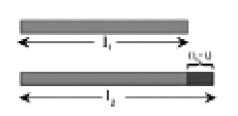Physics

# What is Expansion of Solids?

With the application of heat the length, area and volume of solid substance expands.

Linear expansion of solid and coefficient of linear expansion:

When heat is applied to a solid substance elongation of its length occurs towards a definite direction and the expansion along its length is called linear expansion.Let the length of a bar at θ1 temperature be l1 and at θ2 temperature the length be 12.

Increase in length = I2 – I1

And increase of temperature = θ2 – θ1

The coefficient of linear expansion is denoted by a and it can be expressed as,

α = (I2 – I1)/ I12 – θ1) … …… ……(1)

= [Increase in length / (Initial length x increase of temperature)]

In equation (1), if the initial length I1 = lm and increase of temperature, θ2 – θ1 = 1K, then  α = (I2 – I1) = increase in length.

So, the increase in length of a solid rod of lm for a rise of temperature 1K is defined as the coefficient of linear expansion of the material of the solid.

Its unit is K-1. The coefficient of linear expansion of copper is 16.7 x 10-6 K-1 means if the temperature of copper rod is raised through 1K, then its length increases by 16.7 x 10-6 m.